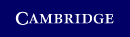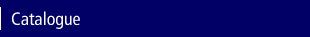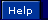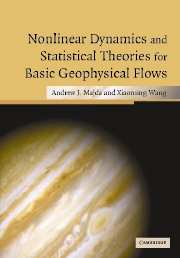Home > Catalogue > Nonlinear Dynamics and Statistical Theories for Basic Geophysical FlowsGoogle Book Search

Search this book

## Details

• 150 b/w illus. 10 tables
• Page extent: 564 pages
• Size: 247 x 174 mm
• Weight: 1.25 kg

## Library of Congress

• Dewey number: 551.01532051
• Dewey version: 22
• LC Classification: QC809.F5 M35 2006
• LC Subject headings:
• Geophysics--Fluid models
• Fluid dynamics
• Fluid mechanics
• Geophysics--Mathematical models
• Statistical mechanics

Library of Congress Record

### Hardback

(ISBN-13: 9780521834414 | ISBN-10: 0521834414)

• Also available in Adobe eBook
• ### For price and ordering options, inspection copy requests, and reading lists please select:

Please note local prices may apply

The general area of geophysical fluid mechanics is truly interdisciplinary. Now ideas from statistical physics are being applied in novel ways to inhomogeneous complex systems such as atmospheres and oceans. In this book, the basic ideas of geophysics, probability theory, information theory, nonlinear dynamics and equilibrium statistical mechanics are introduced and applied to large time-selective decay, the effect of large scale forcing, nonlinear stability, fluid flow on a sphere and Jupiter's Great Red Spot. The book is the first to adopt this approach and it contains many recent ideas and results. Its audience ranges from graduate students and researchers in both applied mathematics and the geophysical sciences. It illustrates the richness of the interplay of mathematical analysis, qualitative models and numerical simulations which combine in the emerging area of computational science.

• First book combining nonlinear dynamical and statistical approaches at elementary level • Only basic prerequisites; many topics introduced through examples as and when required • Many applications to geophysics including Great Red Spot of Jupiter

### Contents

1. Barotropic geophysical flows and two-dimensional fluid flows: an elementary introduction; 2. The Response to large scale forcing; 3. The selective decay principle for basic geophysical flows; 4. Nonlinear stability of steady geophysical flows; 5. Topographic mean-flow interaction, nonlinear instability, and chaotic dynamics; 6. Introduction to empirical statistical theory; 7. Equilibrium statistical mechanics for systems of ordinary differential equations; 8. Statistical mechanics for the truncated quasi-geostrophic equations; 9. Empirical statistical theories for most probable states; 10. Assessing the potential applicability of equilibrium statistical theories for geophysical flows: an overview; 11. Predictions and comparison of equilibrium statistical theories; 12. Equilibrium statistical theories and dynamical modeling of flows with forcing and dissipation; 13. Predicting the jets and spots on Jupiter by equilibrium statistical mechanics; 14. Statistically relevant and irrelevant conserved quantities for truncated quasi-geostrophic flow and the Burger–Hopf model; 15. A mathematical framework for quantifying predictability utilizing relative entropy; 16. Barotropic quasi-geostrophic equations on the sphere; Bibliography; Index.

### Review

'… this book is a valuable contribution to the fascinating intersection of applied mathematics and geophysical fluid dynamics. … The authors are adept at illuminating and motivating rigorous mathematical analysis, qualitative models and physical intuition through exceptionally lucid exposition and a rich collection of examples.' Mathematical Reviews